Courses

# Test: Periodic Properties- 2

## 30 Questions MCQ Test Inorganic Chemistry | Test: Periodic Properties- 2

Description
This mock test of Test: Periodic Properties- 2 for Chemistry helps you for every Chemistry entrance exam. This contains 30 Multiple Choice Questions for Chemistry Test: Periodic Properties- 2 (mcq) to study with solutions a complete question bank. The solved questions answers in this Test: Periodic Properties- 2 quiz give you a good mix of easy questions and tough questions. Chemistry students definitely take this Test: Periodic Properties- 2 exercise for a better result in the exam. You can find other Test: Periodic Properties- 2 extra questions, long questions & short questions for Chemistry on EduRev as well by searching above.
QUESTION: 1

Solution:
QUESTION: 2

Solution:
QUESTION: 3

### Consider the following changes: M (s) → M (g)     ........(1) M (s) → M2+ (g) + 2e-   .......(2) M (g) → M+ (g) + 2e-   .......(3) M+ (g) → M2+ (g) + e-  .......(4) M (g) → M2+ (g) + 2e- .......(5)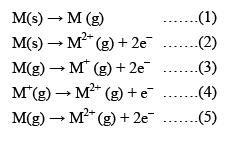The second ionization energy of M could be calculated from the energy values associated with:

Solution:

M (s) → M (g)     ........(1)
M (s) → M2+ (g) + 2e-   .......(2)
M (g) → M+ (g) + 2e-   .......(3)
M+ (g) → M2+ (g) + e-  .......(4)
M (g) → M2+ (g) + 2e- .......(5)

As we are interested in 2nd I.E.
i.e. Energy required to form M2+ (g) from M+ (g)
∴ 4 is correct.
but this option doesn't exist.
⇒ 1 + 3 + 4 is also wrong
1 + 5 gives total energy for M(g) → M2+ (g)
∴ it is also wrong.
3rd reaction is wrong,
∴ 2 - 1 + 3 also dismised.
⇒ (d) is correct

QUESTION: 4

The correct order of second I. E. of C, N, O and F are in the order:

Solution:
QUESTION: 5

Which is the correct order of ionization energies?

Solution:
QUESTION: 6

Which of the following statements is incorrect:

Solution:
QUESTION: 7

First ionization energy is the lowest with:

Solution:
QUESTION: 8

The incorrect statement among the following is:

Solution:

I.E.2 of Na is higher than that of Mg because in case in Na, the second e has to be removed from the noble gas core while in case of magnesium removal of second e- electron gives a noble gas core. Mg has higher first ionization potential than Na because of its stable ns2 configuration.

QUESTION: 9

The correct values of ionization enthalpies (in kJ mol–1) of Si, P, Cl and S respectively are:

Solution:
QUESTION: 10

The third ionization energy is maximum for:

Solution:

Nitrogen has highest third ionization energy due to charge density.  As when the three electrons are remove from the gaseous state then boron have fully filled orbital that's why more energy is needed to remove the electron so more is ionisation energy.

QUESTION: 11

Consider the following changes
A → A+ + e- : E1
and A+ → A2+ + e- ; E2
The energy required to pull out the two electrons are E1 and E2 respectively. The correct relationship between two energies would be...

Solution:
QUESTION: 12

The incorrect statement is:

Solution:
QUESTION: 13

First the ionization energies (in kJ/mol) of three representative elements are given below: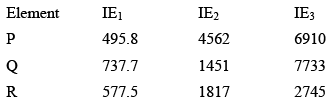Then incorrect option is:

Solution:
QUESTION: 14

Which of the following statement is correct regarding following process: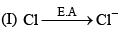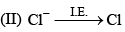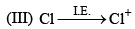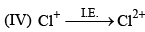Solution:
QUESTION: 15

The correct order of increasing electron affinity of the following elements is:

Solution:

Electron affinity generally becomes more negative on moving from left to right along a period. It is because on moving across a period, the atomic size decreases.

As a result, the force of attraction exerted by the nucleus on the electron increases.

Consequently, the atom has a greater tendency to gain an electron. Hence, electron gain enthalpy becomes more negative.

Cl has more negative electron gain enthalpy than fluorine because in it the added electron goes to the smaller energy shell(n=2) and hence suffers significant repulsion from the electrons already present in this shell. And fluorine also possesses high charge density.

QUESTION: 16

The second electron gain enthalpies (in kJ mol–1) of oxygen and sulphur respectively are:

Solution:
QUESTION: 17

Which of the following statements is correct:

Solution:
QUESTION: 18

Which one of the following statements is incorrect:

Solution:

Electron gain enthalpy is the energy released when an electron is added to an isolated gaseous atom.

Its value is negative and the ease with which an atom accepts electrons its electron gain enthalpy becomes more negative that is, its value increases.

With an increase in nuclear charge, the electron gain enthalpy also increases.

Due to the extra stability of nitrogen (it has half-filled valence shell [He]1s22p3, which provides extra stability to it), it is very difficult to add an electron to it.

Therefore, it has almost zero electron gain enthalpy.

In general, on moving down the group, the electron gain enthalpy decreases due to an increase in the atomic size, but on moving from F to Cl the electron gain enthalpy increases.

Due to the very small size of F atom and absence of 3rd shell, the incoming electron experiences repulsion due to the valence electrons and its electron gain enthalpy is less than Cl. After Cl the electron gain enthalpy follows the general order and decreases on moving down to Br and I.

QUESTION: 19

The formation of the oxide ion O2–(g) requires first an exothermic and then an endothermic step as shown below: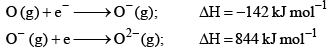This is because:

Solution:
QUESTION: 20

Identify the wrong sequence of the elements in a group

Solution:
QUESTION: 21

The electron affinity of the following elements can be arranged:

Solution:

Electron affinity represents tendency of an atom to accept an electron. Electron affinity increases from left to right and decreases down the group. The images attached compares the electron affinity values of elements.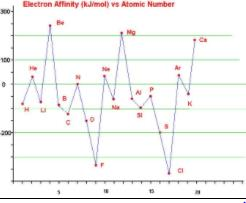QUESTION: 22

In which of the following arrangements, the order is not correct according to the property indicated against it:

Solution:
QUESTION: 23

Which of the following statements is/are wrong:

Solution:

All isoelectronic ions belong to the same period of the periodic table, is a wrong statement as F− and Na+ are isoelectronic but they belong to different periods.

QUESTION: 24

Consider the following conversions: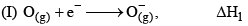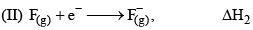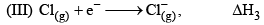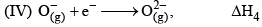That according to given informat ion the incorrect statement is:

Solution:
QUESTION: 25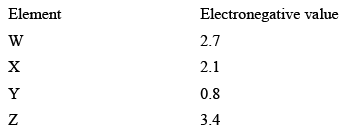The incorrect statement regarding given information is

Solution:
QUESTION: 26

In the compound M—O—H, the M—O bond will be broken if:

Solution:
QUESTION: 27

An element has an atomic number of 15 with which of the following elements will it show similar chemical properties.

Solution:
QUESTION: 28

If the ionization and electron gain enthalpy of an element are 275 and 86 Kcalmol−1 respectively, then the electronegativity of the element on the Mulliken scale is:

Solution: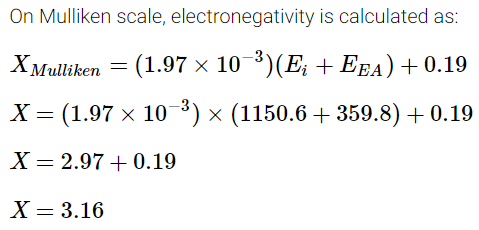QUESTION: 29

Consider the following statements: (I) The radius of an anion is larger than that of the parent atom. (II) The ionization energy generally increases with increasing atomic number in a period (III) The electronegativity of an element is the tendency of an isolated atom to attract an electron.Which of the above statements is/are correct:

Solution:
QUESTION: 30

Which of the following order is correct for the property ment ioned in brackets:

Solution:

Track your progress, build streaks, highlight & save important lessons and more!

### Similar Content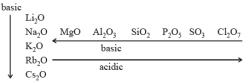### Related tests